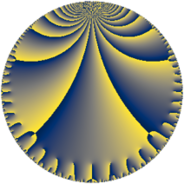# Properties

 Label 152.7.eLevel $152$ Weight $7$ Character orbit 152.e Rep. character $\chi_{152}(113,\cdot)$ Character field $\Q$ Dimension $30$ Newform subspaces $1$ Sturm bound $140$ Trace bound $0$

# Related objects

## Defining parameters

 Level: $$N$$ $$=$$ $$152 = 2^{3} \cdot 19$$ Weight: $$k$$ $$=$$ $$7$$ Character orbit: $$[\chi]$$ $$=$$ 152.e (of order $$2$$ and degree $$1$$) Character conductor: $$\operatorname{cond}(\chi)$$ $$=$$ $$19$$ Character field: $$\Q$$ Newform subspaces: $$1$$ Sturm bound: $$140$$ Trace bound: $$0$$

## Dimensions

The following table gives the dimensions of various subspaces of $$M_{7}(152, [\chi])$$.

Total New Old
Modular forms 124 30 94
Cusp forms 116 30 86
Eisenstein series 8 0 8

## Trace form

 $$30q - 720q^{7} - 8670q^{9} + O(q^{10})$$ $$30q - 720q^{7} - 8670q^{9} - 2524q^{11} + 9700q^{17} + 4014q^{19} - 39376q^{23} + 110742q^{25} - 19976q^{35} - 266500q^{39} - 106788q^{43} - 91360q^{45} + 222756q^{47} + 593586q^{49} + 540936q^{55} - 545972q^{57} - 242640q^{61} - 377716q^{63} + 545964q^{73} - 272356q^{77} + 2189926q^{81} + 1542652q^{83} - 826908q^{85} - 2729572q^{87} - 2139912q^{93} + 2142716q^{95} - 293012q^{99} + O(q^{100})$$

## Decomposition of $$S_{7}^{\mathrm{new}}(152, [\chi])$$ into newform subspaces

Label Dim. $$A$$ Field CM Traces $q$-expansion
$$a_2$$ $$a_3$$ $$a_5$$ $$a_7$$
152.7.e.a $$30$$ $$34.968$$ None $$0$$ $$0$$ $$0$$ $$-720$$

## Decomposition of $$S_{7}^{\mathrm{old}}(152, [\chi])$$ into lower level spaces

$$S_{7}^{\mathrm{old}}(152, [\chi]) \cong$$ $$S_{7}^{\mathrm{new}}(19, [\chi])$$$$^{\oplus 4}$$$$\oplus$$$$S_{7}^{\mathrm{new}}(38, [\chi])$$$$^{\oplus 3}$$$$\oplus$$$$S_{7}^{\mathrm{new}}(76, [\chi])$$$$^{\oplus 2}$$